Courses

# Equation Of The Gas State Processes (Part - 1) - Heat, Irodov JEE Notes | EduRev

## NEET : Equation Of The Gas State Processes (Part - 1) - Heat, Irodov JEE Notes | EduRev

The document Equation Of The Gas State Processes (Part - 1) - Heat, Irodov JEE Notes | EduRev is a part of the NEET Course I. E. Irodov Solutions for Physics Class 11 & Class 12.
All you need of NEET at this link: NEET

Q. 1. A vessel of volume V = 30 l contains ideal gas at the temperature 0°C. After a portion of the gas has been let out, the pressure in the vessel decreased by Δp = 0.78 atm (the temperature remaining constant). Find the mass of the released gas. The gas density under the normal conditions p = 1.3 g/I.

Solution. 1. Let mand m2 be the masses of the gas in the vessel before and after the gas is released. Hence mass of the gas released,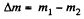Now from ideal gas equation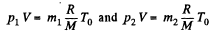as V and T are same before and after the release of the gas.

so,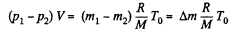or,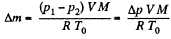(1)

We also know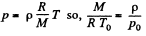(2)

(where p0 = standard atmospheric pressure and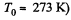From Eqs. (1) and (2) we get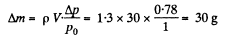Q. 2. Two identical vessels are connected by a tube with a valve letting the gas pass from one vessel into the other if the pressure difference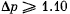atm. Initially there was a vacuum in one vessel while the other contained ideal gas at a temperature t1  = 27°C and pressure p1  = 1.00 atm. Then both vessels were heated to a temperature t2 =107°C. Up to what value will the pressure in the first vessel (which had vacuum initially) increase?

Solution. 2. Let m1 be the mass of the gas enclosed.

Then,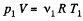When heated, some gas, passes into the evacuated vessel till pressure difference becomes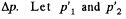be the pressure on the two sides of the valve. Then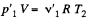and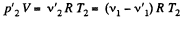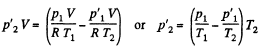But,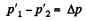So,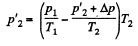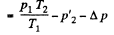or,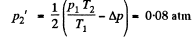Q. 3. A vessel of volume V = 20 l contains a mixture of hydrogen and helium at a temperature t = 20°C and pressure p = 2.0 atm. The mass of the mixture is equal to m = 5.0 g. Find the ratio of the mass of hydrogen to that of helium in the given mixture.

Solution. 3. Let the mixture contain v1 and v2 moles of H2 and He respectively. If molecular weights of H2 and He are M1 and M2, then respective masses in the mixture are equal to

m1 = v1M1 and m2 = v2M2

Therefore, for the total mass of the mixture we get,

m = m1 + m2 or m = v1 M1 + v2 M2           (1)

Also, if v is the total number of moles of the mixture in the vessels, then we know,

V = V1 + V2                  (2)

Solving (1) and (2) for v1 and v2, we get,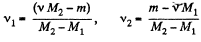Therefore, we get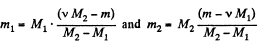or,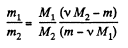One can also express the above result in terms of the effective molecular weight M of the mixture, defined as,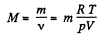Thus,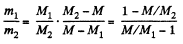Using the data and table, we get :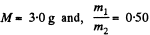Q. 4. A vessel contains a mixture of nitrogen (m1 =  7.0 g) and carbon dioxide (m2  = 11 g) at a temperature T = 290 K and pressure p0, = 1.0 atm. Find the density of this mixture, assuming the gases to be ideal.

Solution. 4. We know, for the mixture, N2 and CO2 (being regarded as ideal gases, their mixture too behaves like an ideal gas)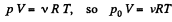where, v is the total number of moles of the gases (mixture) present and V is the volume of the vessel. If v1 and v2 are number of moles of N2 and CO2 respectively present in the mixture, then

v = v1 + v2

Now number of moles of N2 and CO2 is, by definition, given by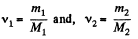where, m1 is the mass of N2 (Moleculer weight = M1) in the mixture and m2 is the mass of CO2 (Molecular weight = M2) in the mixture.
Therefore density of the mixture is given by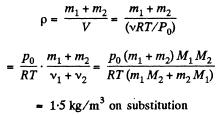Q. 5. A vessel of volume V = 7.5 1 contains a mixture of ideal gases at a temperature T = 300 K: v1 =  0.10 mole of oxygen, v2 = 0.20 mole of nitrogen, and v3 = 0.30 mole of carbon dioxide. Assuming the gases to be ideal, find:
(a) the pressure of the mixture;
(b) the mean molar mass M of the given mixture which enters its equation of state pV = (m/M) RT, where m is the mass of the mixture.

Solution. 5. (a) The mixture contains v1, v2 and v3 moles of O> N2 and CO2 respectively. Then the total number of moles of the mixture

v = v1 + v2 + v3

We know, ideal gas equation for the mixture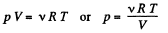or,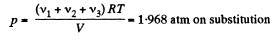(b ) M ass o f oxygen (O2) present in the mixture : m1 = v1 M1

Mass of nitrogen (N2) present in the mixture : m2 = v2M2

Mass of carbon dioxide (CO2) present in the mixture : m3 = v3M3

So, mass of the mixture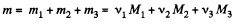Moleculer mass of the mixture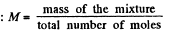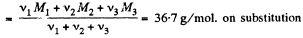Q. 6. A vertical cylinder closed from both ends is equipped with an easily moving piston dividing the volume into two parts, each containing one mole of air. In equilibrium at T0 = 300 K the volume of the upper part is η = 4.0 times greater than that of the lower part. At what temperature will the ratio of these volumes be equal η' = 3.0?

Solution. 6. Let p1 and p2 be the pressure in the upper and lower part of the cylinder respectively at temperature T0. At the equilibrium position for the piston :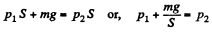(m is the mass o f the piston.)

But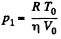(where V0 is the initial volume of the lower part)

So,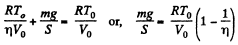(1)

Let T be the sought temperature and at this temperature the volume of the lower part becomes V, then according to the problem the volume of the upper part becomes η'V'

Hence,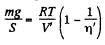(2)

From (1) and (2).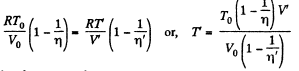As, the total volume must be constant,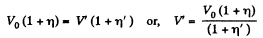Putting the value of V in Eq. (3), we get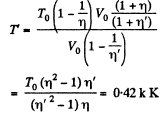Q. 7. A vessel of volume V is evacuated by means of a piston air pump. One piston stroke captures the volume ΔV. How many strokes are needed to reduce the pressure in the vessel η times? The process is assumed to be isothermal, and the gas ideal.

Solution. 7. Let P1 be the density after the first stroke. The the mass remains constant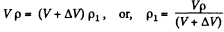Similarly, if p2 is the density after second stroke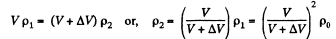In this way after nth stroke.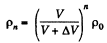Since pressure α density,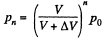(because temperature is constant)

It is required by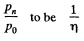so,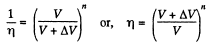Hence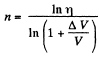Q. 8. Find the pressure of air in a vessel being evacuated as a function of evacuation time t. The vessel volume is V, the initial pressure is P0. The process is assumed to be isothermal, and the evacuation rate equal to C and independent of pressure.

Note. The evacuation rate is the gas volume being evacuated per unit time, with that volume being measured under the gas pressure attained by that moment.

Solution. 8. From the ideal gas equation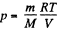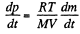(1)

In each stroke, volume v of the gas is ejected, where v is given by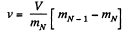In case of continuous ejection, if (mN-1) corresponds to mass of gas in the vessel at time t, then mN is the mass at time t + Δt, where Δt, is the time in which volume v of the ga

has come out. The rate of evacuation is therefore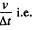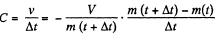In the lim it Δt - 0, we get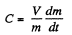(2)

From (1) and (2)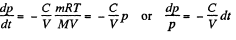Integrating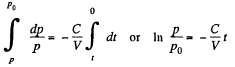Thus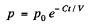Q. 9. A chamber of volume V = 87 l is evacuated by a pump whose evacuation rate (see Note to the foregoing problem) equals C = 10 1/s. How soon will the pressure in the chamber decrease by η = 1000 times?

Solution. 9. Let p be the instantaneous density, then instantaneous mass = Vp. In a short interval dt the volume is increased by Cdt.

So,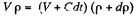(because mass remains constant in a short interval dt)

so,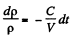Since pressure α density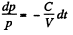or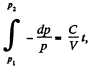or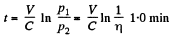Q. 10. A smooth vertical tube having two different sections is open from both ends and equipped with two pistons of different areas (Fig. 2.1). Each piston slides within a respective tube section. One mole of ideal gas is enclosed between the pistons tied with a non-stretchable thread. The crosssectional area of the upper piston is ΔS = 10 cm2 greater than that of the lower one. The combined mass of the two pistons is equal to m = 5.0 kg. The outside air pressure is P0  = 1.0 atm. By how many kelvins must the gas between the pistons be heated to shift the pistons through l = 5.0 cm?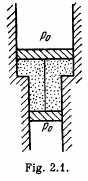Solution. 10. The physical system consists of one mole of gas confined in the smooth vertical tube. Let m1 and m2 be the masses of upper and lower pistons and S1 and S2 are their respective areas.

For the lower piston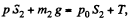or,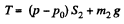(1)

Similarly for the upper piston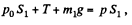or,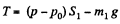(2)

From (1) and (2)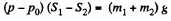or,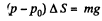so,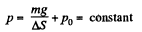From the gas law, p V = v R T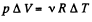(because p is constant)

So,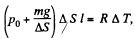Hence,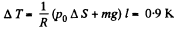Q. 11. Find the maximum attainable temperature of ideal gas in each of the following processes: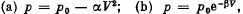where p0,,α and β are positive constants, and V is the volume of one mole of gas.

Solution. 11.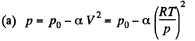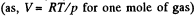Thus,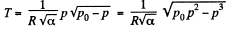(1)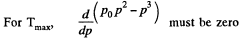which yields,(2)

Hence,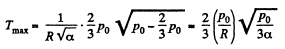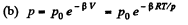so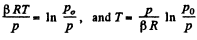(1)

For Tmax the condition is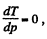which yields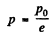Hence using this value of p in Eq. (1), we get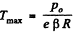Q. 12. Find the minimum attainable pressure of ideal gas in the process T = T0  + α V2, where T0  and α are positive constants, and V is the volume of one mole of gas. Draw the approximate p vs V plot of this process.

Solution. 12.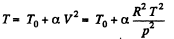(as, V = RT/p for one mole of gas)

So,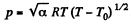(1)

For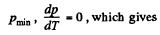T = 2 T0     (2)

From (1) and (2), we get,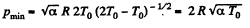Offer running on EduRev: Apply code STAYHOME200 to get INR 200 off on our premium plan EduRev Infinity!

111 docs

,

,

,

,

,

,

,

,

,

,

,

,

,

,

,

,

,

,

,

,

,

,

,

,

;